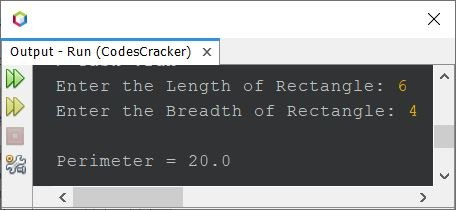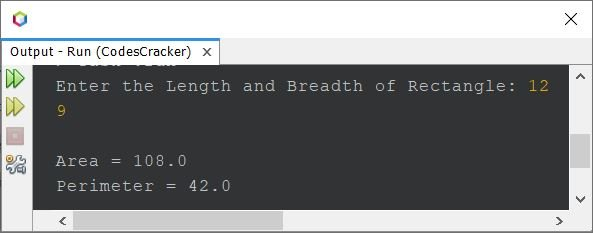# Java Program to Calculate Area and Perimeter of a Rectangle

This article covers a program in Java that find and prints area and perimeter of a rectangle based on its size provided by user at run-time of the program.

Note - The area of a rectangle is calculated using the formula len*bre. Where len and bre are the values of length and breadth, the two adjacent sides of rectangle, where length is greater than breadth.

Note - The perimeter of a rectangle is calculated using the formula (2*len) + (2*bre) or 2*(len+bre).

## Find Area of Rectangle in Java

The question is, write a program in Java that calculates area of rectangle. The program given below is its answer:

```import java.util.Scanner;

public class CodesCracker
{
public static void main(String[] args)
{
float len, bre, area;
Scanner s = new Scanner(System.in);

System.out.print("Enter the Length of Rectangle: ");
len = s.nextFloat();
System.out.print("Enter the Breadth of Rectangle: ");
bre = s.nextFloat();

area = len*bre;
System.out.println("\nArea = " +area);
}
}```

The snapshot given below shows the sample run of above Java program with user input 6 as length and 4 as breadth of rectangle:## Find Perimeter of Rectangle in Java

The question is, write a Java program to find and print perimeter of a rectangle. The answer to this question is the program given below:

```import java.util.Scanner;

public class CodesCracker
{
public static void main(String[] args)
{
Scanner s = new Scanner(System.in);

System.out.print("Enter the Length of Rectangle: ");
length = s.nextFloat();
System.out.print("Enter the Breadth of Rectangle: ");

System.out.println("\nPerimeter = " +perimeter);
}
}```

The sample run of above program with same user input as of previous program's sample run, is shown in the snapshot given below:## Find Area and Perimeter of Rectangle in Java - Single Program

This is the last program of this article, created basically to print area and perimeter both, of a rectangle, whose length and breadth will get entered by user at run-time of the program:

```import java.util.Scanner;

public class CodesCracker
{
public static void main(String[] args)
{
Scanner s = new Scanner(System.in);

System.out.print("Enter the Length and Breadth of Rectangle: ");
float a = s.nextFloat();
float b = s.nextFloat();

float ar = a*b;
float pr = 2*(a+b);
System.out.println("\nArea = " +ar);
System.out.println("Perimeter = " +pr);
}
}```

Here is its sample run with user input 12 as length and 9 as breadth of rectangle:Note - For Area and Perimeter of Square Program in Java, refer to its separate article.

#### Same Program in Other Languages

Java Online Test

« Previous Program Next Program »